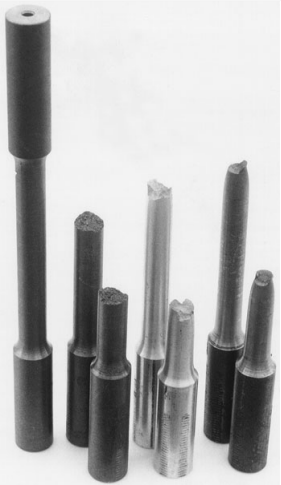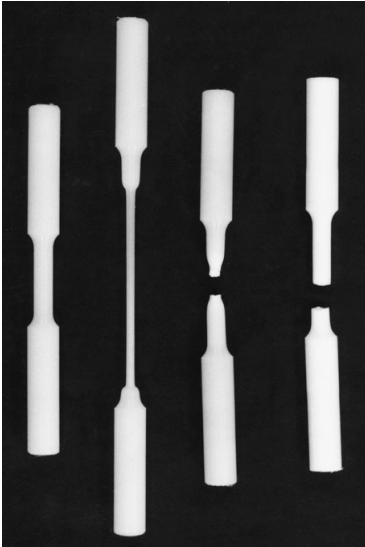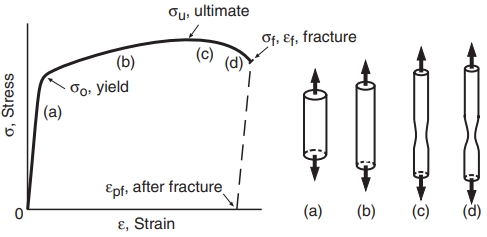A tension test consists of slowly pulling a sample of material with an axial force until it breaks. The test specimen used may have either a circular or a rectangular cross section, and its ends are usually enlarged to provide extra area for gripping and to avoid having the sample break where it is being gripped. Specimens both before and after testing are shown for several metals and polymers in Figs. 1 and 2.Figure 1 Tensile specimens of metals (left to right): untested specimen with 9 mm diameter test section, and broken specimens of gray cast iron, aluminum alloy 7075-T651, and hot-rolled AISI 1020 steel.Figure 2 Tensile specimens of polymers (left to right): untested specimen with a 7.6 mmdiameter test section, a partially tested specimen of high-density polyethylene (HDPE), and broken specimens of nylon 101 and Teflon (PTFE).

Methods of gripping the ends vary with specimen geometry. A typical arrangement for threaded-end specimens is shown in Fig. 3.

Note that spherical bearings are used at each end to provide a pure tensile force, with no undesirable bending. The usual manner of conducting the test is to deform the specimen at a constant speed. For example, in the universal testing machines the motion between the fixed and moving crossheads can be controlled at a constant speed. Hence, distance h in Fig. 3 is varied so that

dh/dt = h = constant

The axial force that must be applied to achieve this displacement rate varies as the test proceeds. This force P may be divided by the cross-sectional area Ai to obtain the stress in the specimen at any time during the test:

σ = P/Ai

Displacements in the specimen are measured within a straight central portion of constant cross section over a gage length Li , as indicated in Fig. 3. Strain ε may be computed from the change in this length,  ΔL:

ε = ΔL/Li

Stress and strain, based on the initial (undeformed) dimensions, Ai and Li , as just presented, are called engineering stress and strain.

It is sometimes reasonable to assume that all of the grip parts and the specimen ends are nearly rigid. In this case, most of the change in crosshead motion is due to deformation within the straight section of the test specimen, so that ΔL is approximately the same as Δh, the change in h.

Strain may therefore be estimated as ε = Δh/Li . However, actual measurement of ΔL is preferable, as use of  Δh may cause considerable error in the measured strain values.

Strain ε as is dimensionless. As a convenience, strains are sometimes given as percentages, where ε% = 100ε. Strains may also be expressed in millionths, called microstrain, where εμ = 106ε.

If strains are given as percentages or as microstrain, then, prior to using the value for most calculations, it is necessary to convert to the dimensionless form ε.

The principal result obtained from a tension test is a graph of engineering stress versus engineering strain for the entire test, called a stress–strain curve. With the use of digital computers in the laboratory, the form of the data is a list of numerical values of stress and strain, as sampled at short time intervals during the test.

Stress–strain curves vary widely for different materials. Brittle behavior in a tension test is failure without extensive deformation. Gray cast iron, glass, and some polymers, such as PMMA (acrylic), are examples of materials with such behavior. A stress–strain curve for gray iron is shown in Fig. 4.

Other materials exhibit ductile behavior, failing in tension only after extensive deformation. Stress–strain curves for ductile behavior in engineering metals and some polymers are similar to Figs. 5 and 6, respectively.Figure 4.9 Schematic of the engineering stress–strain curve of a typical ductile metal that exhibits necking behavior. Necking begins at the ultimate stress point.

One might ask why we describe tension test results in terms of stress and strain, σ and ε, rather than simply force and length change, P and ΔL. Note that samples of a given material with different cross-sectional areas Ai will fail at higher forces for larger areas. By calculating the force per unit area, or stress, this effect of sample size is removed.

Hence, a given material is expected to have the same yield, ultimate, and fracture stress for any cross-sectional area Ai , while the corresponding forces P vary with Ai . (An actual experimental comparison for different Ai will be affected by minor variations in properties with location in the parent batch of material, lack of absolute precision in the laboratory measurements, and other such statistical errors.)

The use of strain ε similarly removes the effect of sample length. For a given stress, specimens with greater length L will exhibit a proportionately larger length change ΔL, but the strain ε corresponding to the yield, ultimate, and fracture points is expected to be the same for any length of sample. Hence, the stress–strain curve is considered to give a fundamental characterization of the behavior of the material.